Скачать презентацию NEW A 2 COMPUTING Intractable Problems Dr K

c242760df3d4b514f3d5ced748079a05.ppt

• Количество слайдов: 40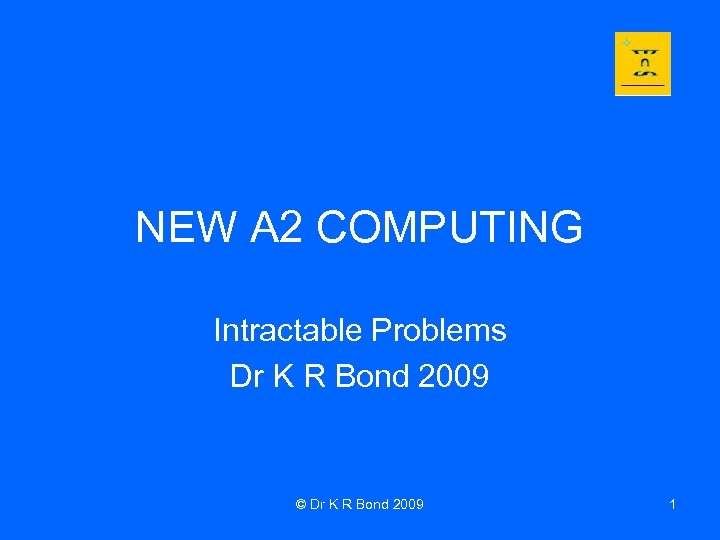NEW A 2 COMPUTING Intractable Problems Dr K R Bond 2009 © Dr K R Bond 2009 1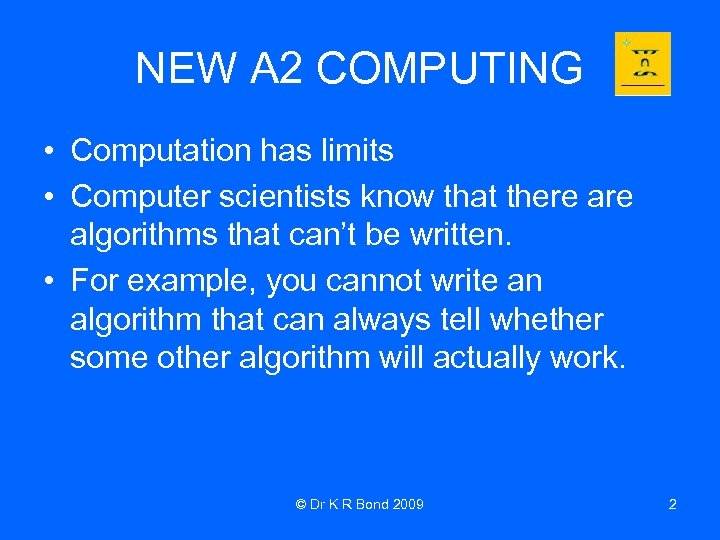NEW A 2 COMPUTING • Computation has limits • Computer scientists know that there algorithms that can’t be written. • For example, you cannot write an algorithm that can always tell whether some other algorithm will actually work. © Dr K R Bond 2009 2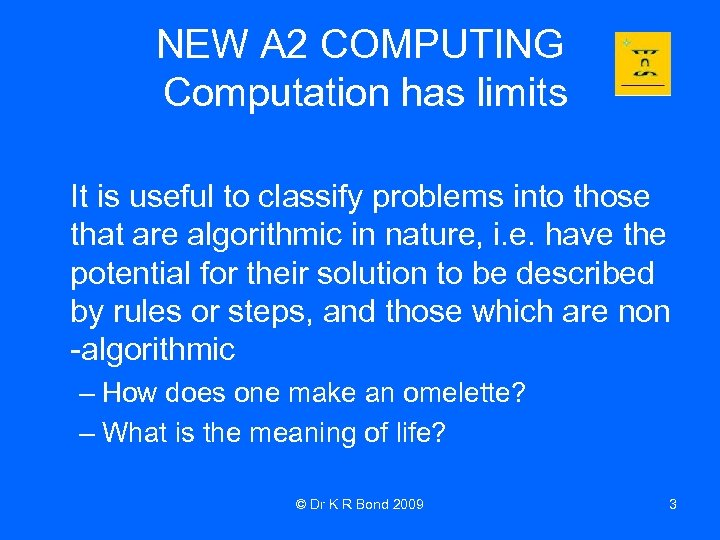NEW A 2 COMPUTING Computation has limits It is useful to classify problems into those that are algorithmic in nature, i. e. have the potential for their solution to be described by rules or steps, and those which are non -algorithmic – How does one make an omelette? – What is the meaning of life? © Dr K R Bond 2009 3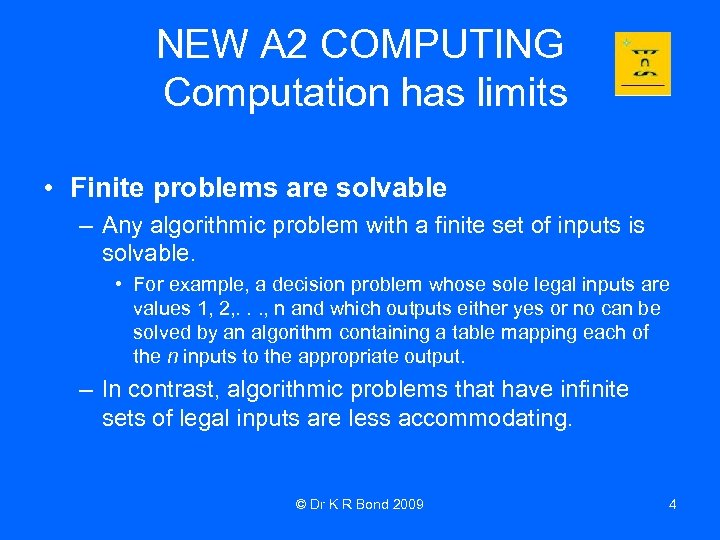NEW A 2 COMPUTING Computation has limits • Finite problems are solvable – Any algorithmic problem with a finite set of inputs is solvable. • For example, a decision problem whose sole legal inputs are values 1, 2, . . . , n and which outputs either yes or no can be solved by an algorithm containing a table mapping each of the n inputs to the appropriate output. – In contrast, algorithmic problems that have infinite sets of legal inputs are less accommodating. © Dr K R Bond 2009 4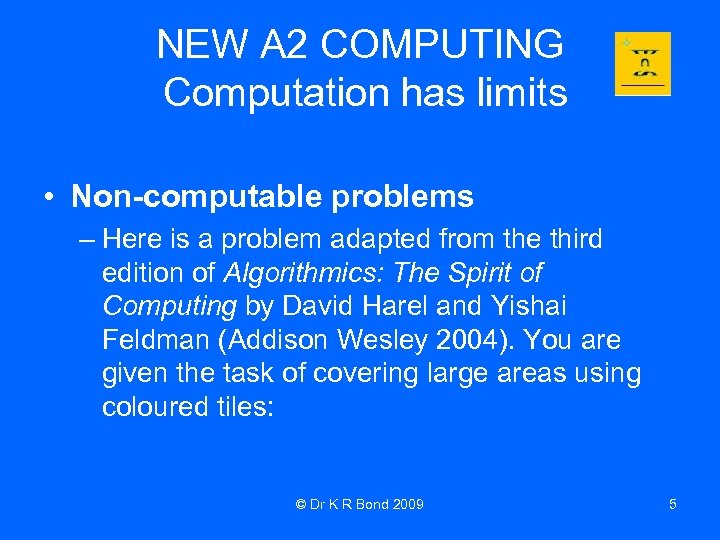NEW A 2 COMPUTING Computation has limits • Non-computable problems – Here is a problem adapted from the third edition of Algorithmics: The Spirit of Computing by David Harel and Yishai Feldman (Addison Wesley 2004). You are given the task of covering large areas using coloured tiles: © Dr K R Bond 2009 5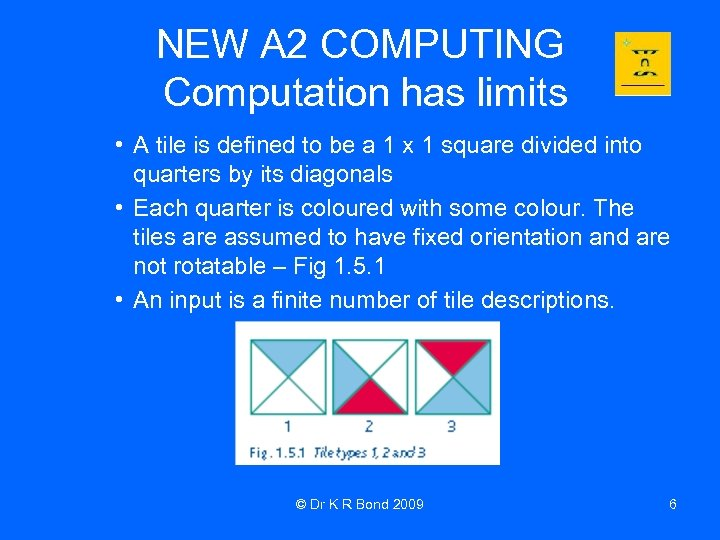NEW A 2 COMPUTING Computation has limits • A tile is defined to be a 1 x 1 square divided into quarters by its diagonals • Each quarter is coloured with some colour. The tiles are assumed to have fixed orientation and are not rotatable – Fig 1. 5. 1 • An input is a finite number of tile descriptions. © Dr K R Bond 2009 6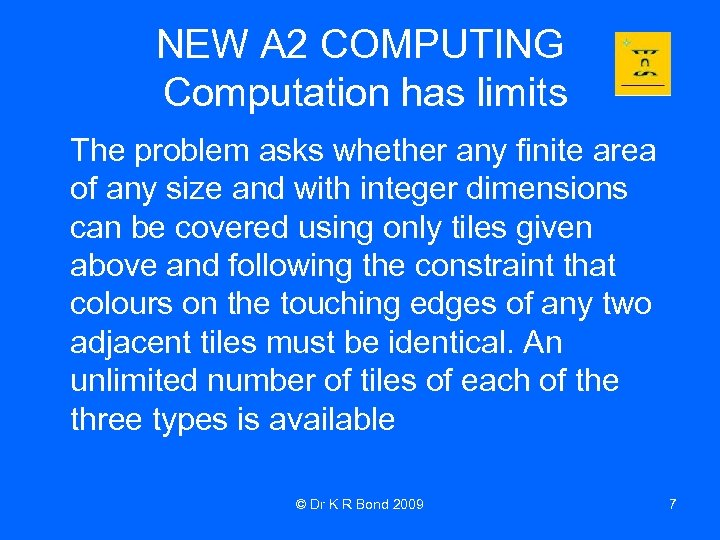NEW A 2 COMPUTING Computation has limits The problem asks whether any finite area of any size and with integer dimensions can be covered using only tiles given above and following the constraint that colours on the touching edges of any two adjacent tiles must be identical. An unlimited number of tiles of each of the three types is available © Dr K R Bond 2009 7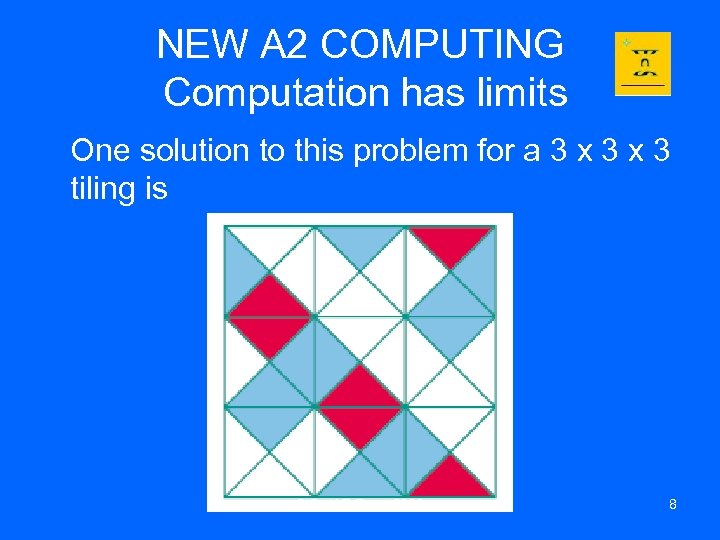NEW A 2 COMPUTING Computation has limits One solution to this problem for a 3 x 3 tiling is © Dr K R Bond 2009 8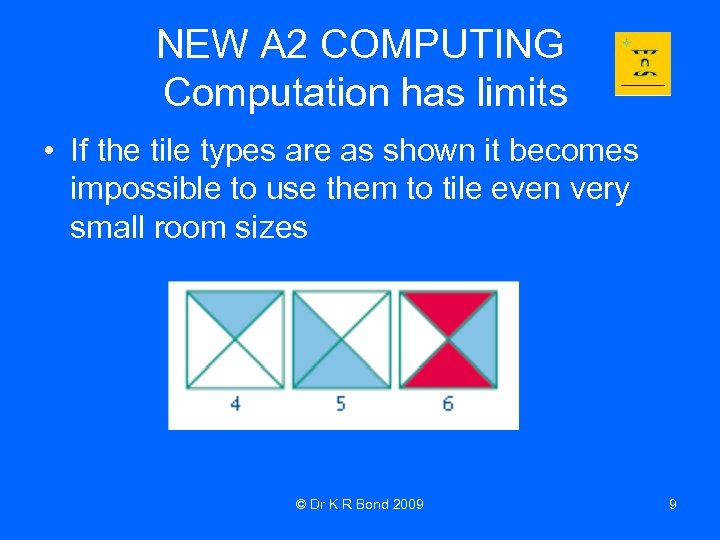NEW A 2 COMPUTING Computation has limits • If the tile types are as shown it becomes impossible to use them to tile even very small room sizes © Dr K R Bond 2009 9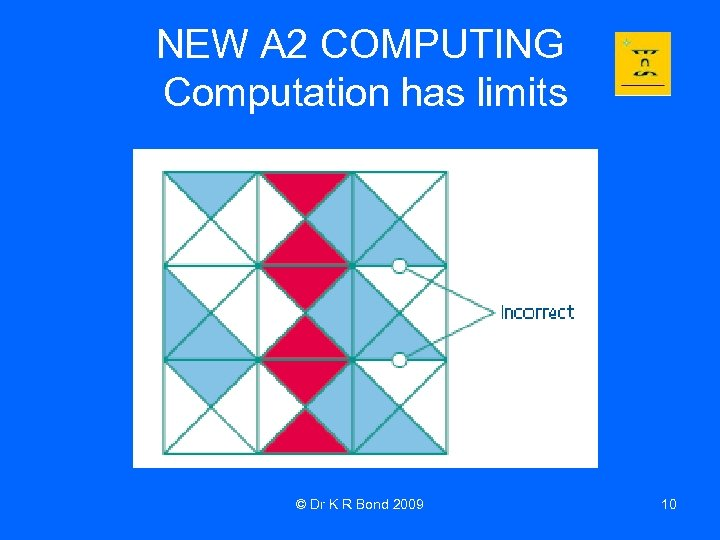NEW A 2 COMPUTING Computation has limits © Dr K R Bond 2009 10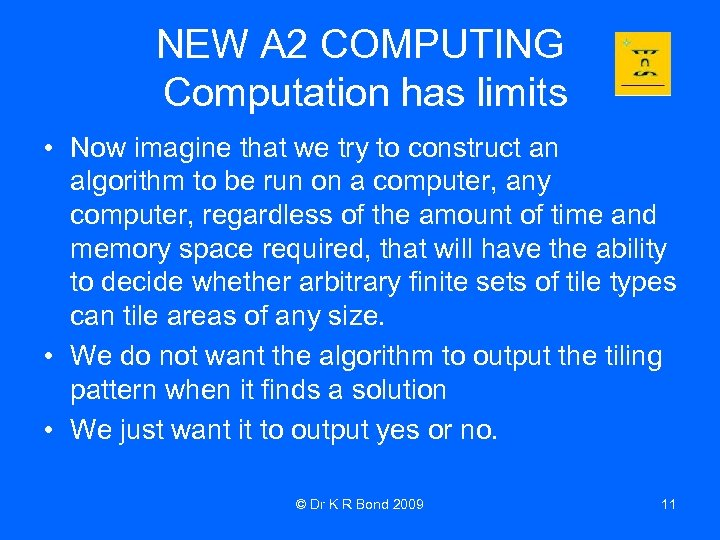NEW A 2 COMPUTING Computation has limits • Now imagine that we try to construct an algorithm to be run on a computer, any computer, regardless of the amount of time and memory space required, that will have the ability to decide whether arbitrary finite sets of tile types can tile areas of any size. • We do not want the algorithm to output the tiling pattern when it finds a solution • We just want it to output yes or no. © Dr K R Bond 2009 11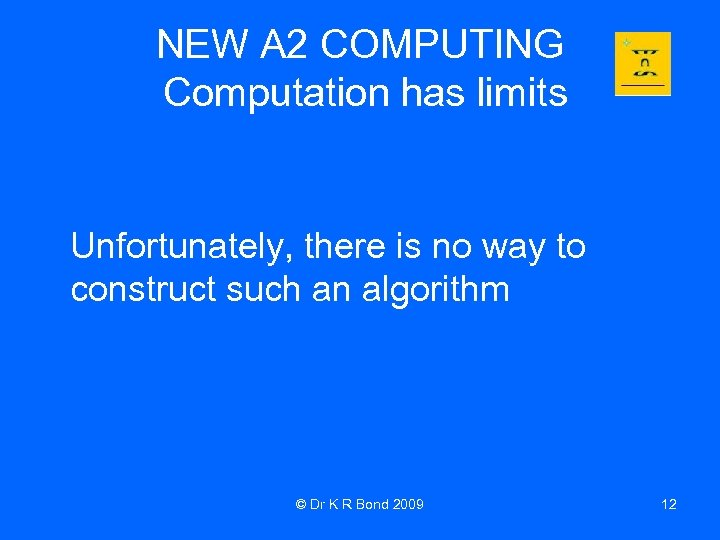NEW A 2 COMPUTING Computation has limits Unfortunately, there is no way to construct such an algorithm © Dr K R Bond 2009 12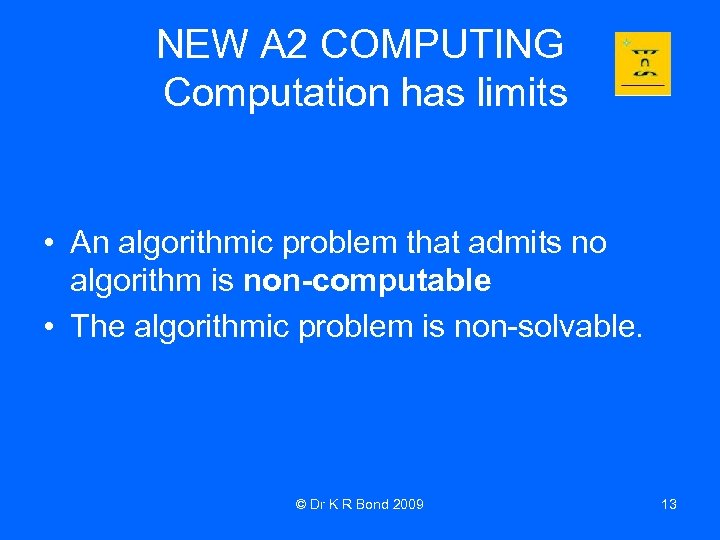NEW A 2 COMPUTING Computation has limits • An algorithmic problem that admits no algorithm is non-computable • The algorithmic problem is non-solvable. © Dr K R Bond 2009 13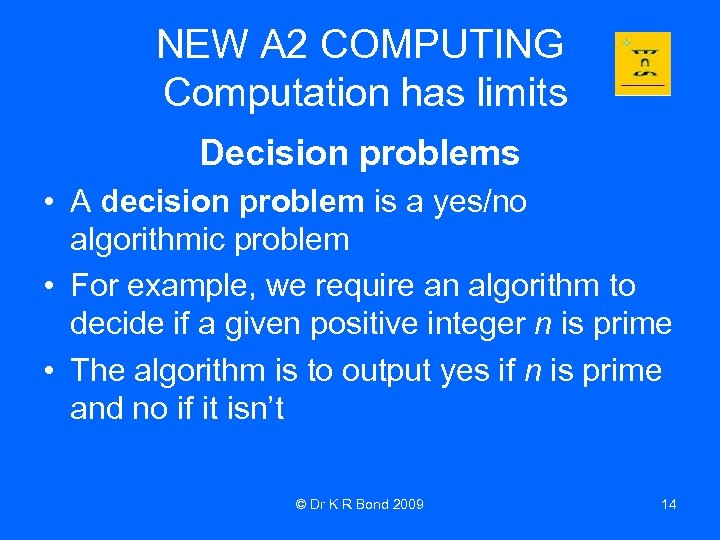NEW A 2 COMPUTING Computation has limits Decision problems • A decision problem is a yes/no algorithmic problem • For example, we require an algorithm to decide if a given positive integer n is prime • The algorithm is to output yes if n is prime and no if it isn’t © Dr K R Bond 2009 14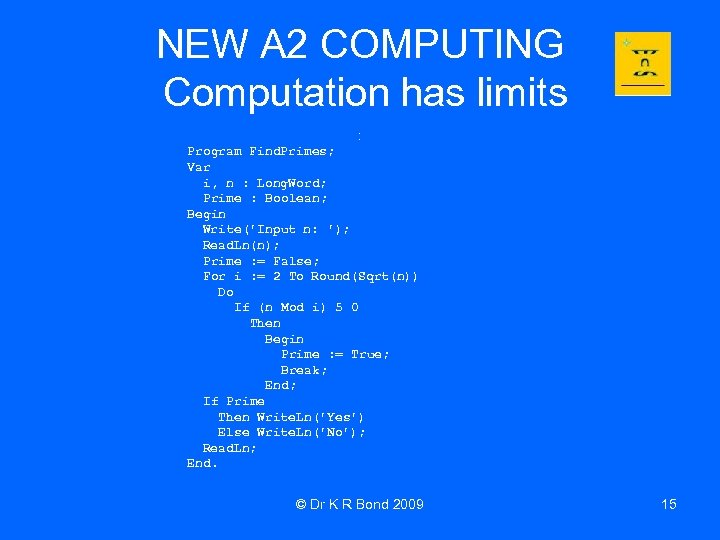NEW A 2 COMPUTING Computation has limits : Program Find. Primes; Var i, n : Long. Word; Prime : Boolean; Begin Write('Input n: '); Read. Ln(n); Prime : = False; For i : = 2 To Round(Sqrt(n)) Do If (n Mod i) 5 0 Then Begin Prime : = True; Break; End; If Prime Then Write. Ln('Yes') Else Write. Ln('No'); Read. Ln; End. © Dr K R Bond 2009 15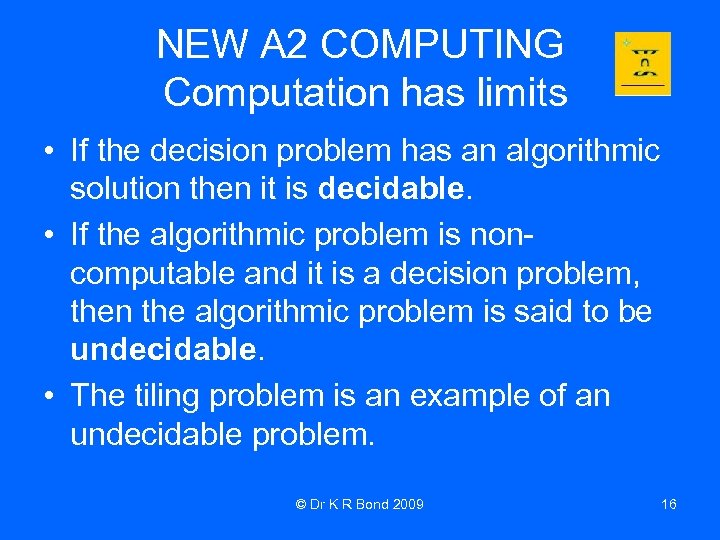NEW A 2 COMPUTING Computation has limits • If the decision problem has an algorithmic solution then it is decidable. • If the algorithmic problem is noncomputable and it is a decision problem, then the algorithmic problem is said to be undecidable. • The tiling problem is an example of an undecidable problem. © Dr K R Bond 2009 16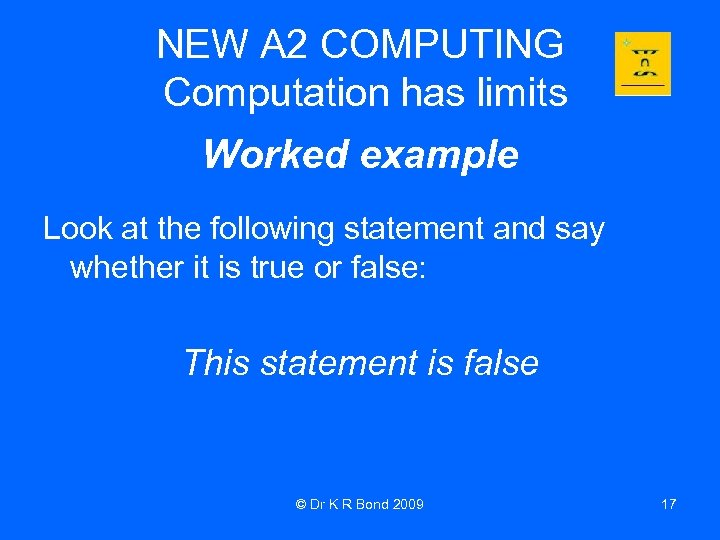NEW A 2 COMPUTING Computation has limits Worked example Look at the following statement and say whether it is true or false: This statement is false © Dr K R Bond 2009 17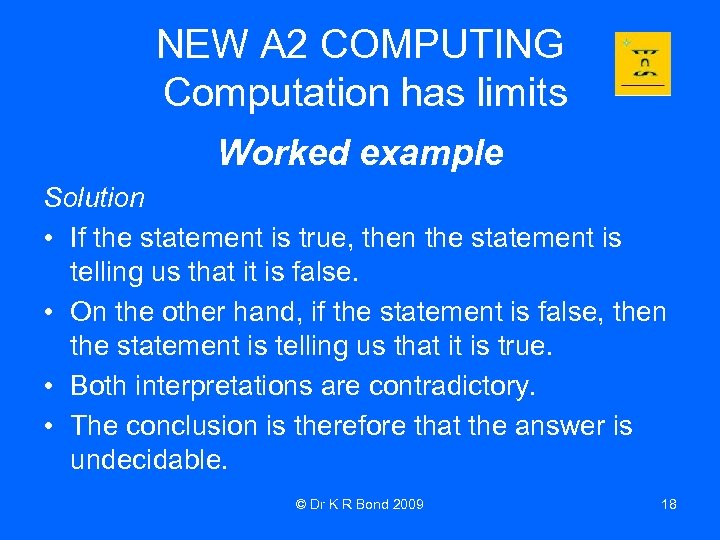NEW A 2 COMPUTING Computation has limits Worked example Solution • If the statement is true, then the statement is telling us that it is false. • On the other hand, if the statement is false, then the statement is telling us that it is true. • Both interpretations are contradictory. • The conclusion is therefore that the answer is undecidable. © Dr K R Bond 2009 18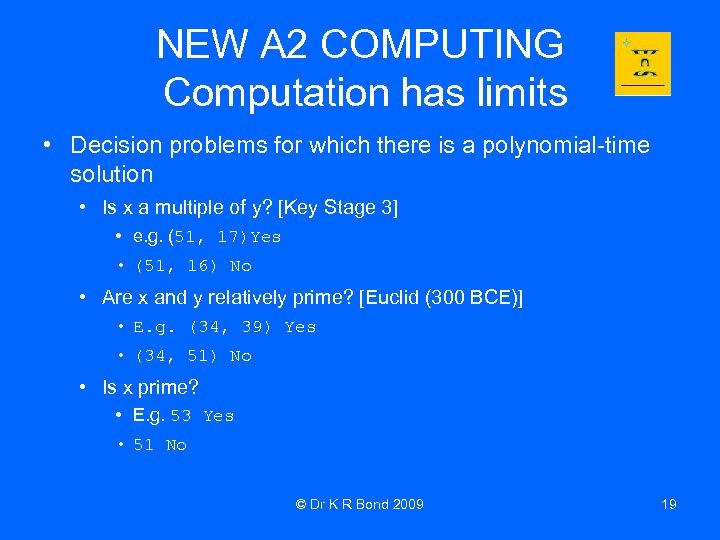NEW A 2 COMPUTING Computation has limits • Decision problems for which there is a polynomial-time solution • Is x a multiple of y? [Key Stage 3] • e. g. (51, 17)Yes • (51, 16) No • Are x and y relatively prime? [Euclid (300 BCE)] • E. g. (34, 39) Yes • (34, 51) No • Is x prime? • E. g. 53 Yes • 51 No © Dr K R Bond 2009 19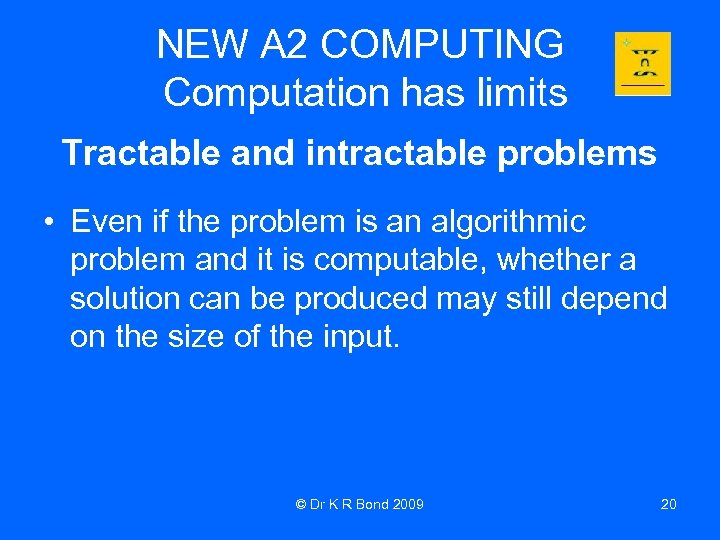NEW A 2 COMPUTING Computation has limits Tractable and intractable problems • Even if the problem is an algorithmic problem and it is computable, whether a solution can be produced may still depend on the size of the input. © Dr K R Bond 2009 20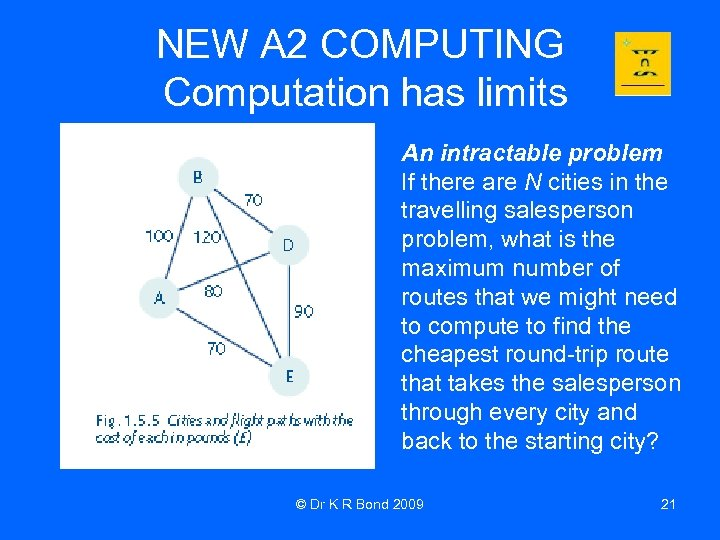NEW A 2 COMPUTING Computation has limits An intractable problem If there are N cities in the travelling salesperson problem, what is the maximum number of routes that we might need to compute to find the cheapest round-trip route that takes the salesperson through every city and back to the starting city? © Dr K R Bond 2009 21NEW A 2 COMPUTING Computation has limits • Pick a starting city • Pick the next city, then N - 1 choices remain Pick the next city, then N - 2 choices remain • Maximum number of routes is (N - 1) * (N - 2) * (N - 3) * …*2*1 • If N = 12 then the maximum number of routes is 39 916 800. • On a medium-speed computer, it will take approximately 39 seconds to evaluate all routes. • If N = 23 then a medium-speed computer will take approximately 51 years. • This is an example of an intractable problem, because the time taken to solve the problem increases dramatically as the number of cities increases. © Dr K R Bond 2009 22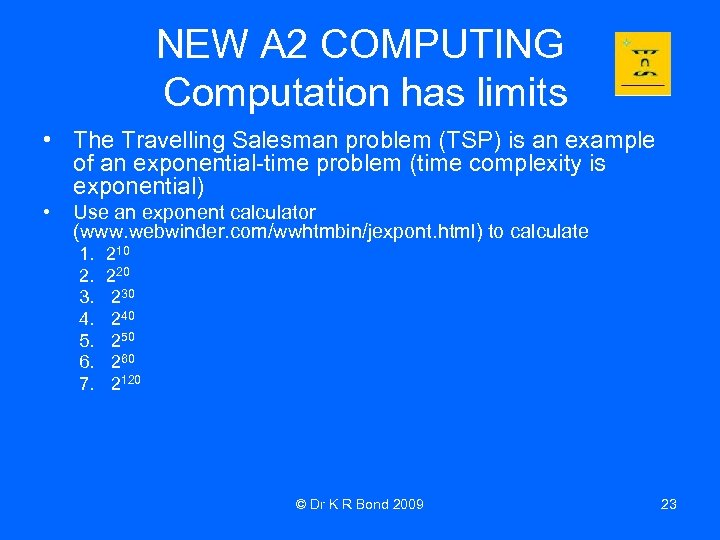NEW A 2 COMPUTING Computation has limits • The Travelling Salesman problem (TSP) is an example of an exponential-time problem (time complexity is exponential) • Use an exponent calculator (www. webwinder. com/wwhtmbin/jexpont. html) to calculate 1. 2. 3. 4. 5. 6. 7. 210 220 230 240 250 260 2120 © Dr K R Bond 2009 23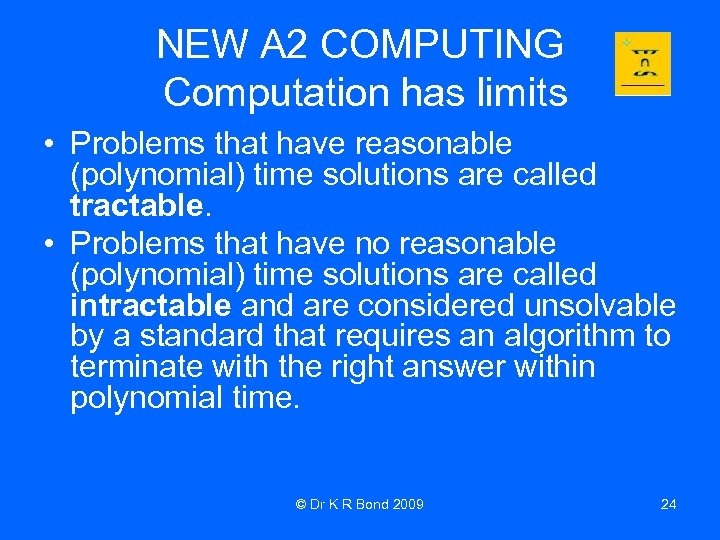NEW A 2 COMPUTING Computation has limits • Problems that have reasonable (polynomial) time solutions are called tractable. • Problems that have no reasonable (polynomial) time solutions are called intractable and are considered unsolvable by a standard that requires an algorithm to terminate with the right answer within polynomial time. © Dr K R Bond 2009 24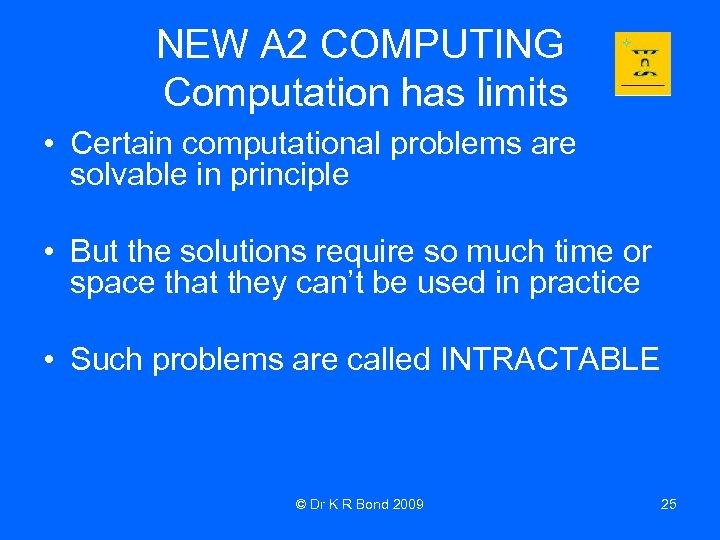NEW A 2 COMPUTING Computation has limits • Certain computational problems are solvable in principle • But the solutions require so much time or space that they can’t be used in practice • Such problems are called INTRACTABLE © Dr K R Bond 2009 25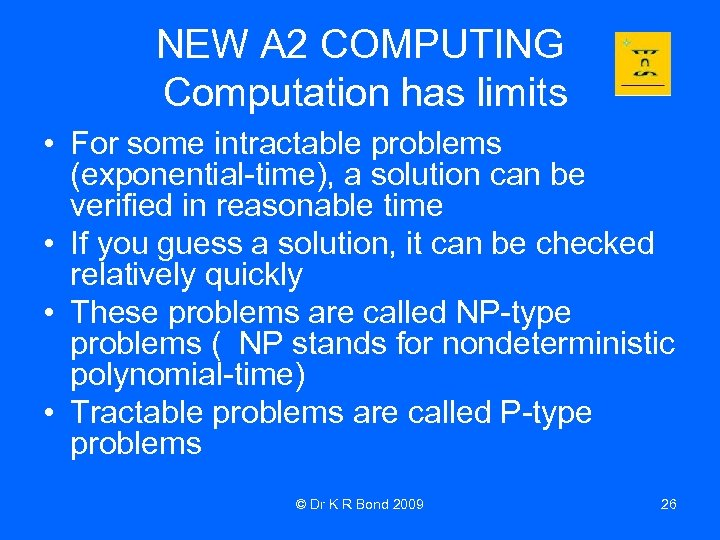NEW A 2 COMPUTING Computation has limits • For some intractable problems (exponential-time), a solution can be verified in reasonable time • If you guess a solution, it can be checked relatively quickly • These problems are called NP-type problems ( NP stands for nondeterministic polynomial-time) • Tractable problems are called P-type problems © Dr K R Bond 2009 26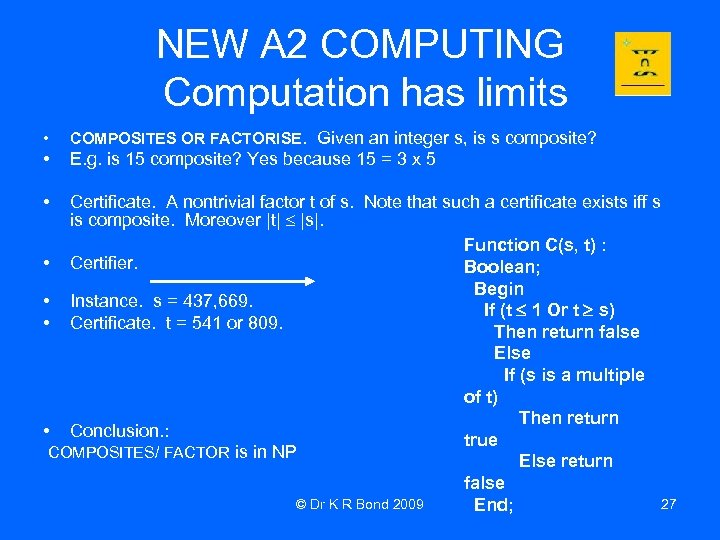NEW A 2 COMPUTING Computation has limits • COMPOSITES OR FACTORISE. Given an integer s, is s composite? • E. g. is 15 composite? Yes because 15 = 3 x 5 • Certificate. A nontrivial factor t of s. Note that such a certificate exists iff s is composite. Moreover |t| |s|. Function C(s, t) : • Certifier. Boolean; Begin • Instance. s = 437, 669. If (t 1 Or t s) • Certificate. t = 541 or 809. Then return false Else If (s is a multiple of t) Then return • Conclusion. : true COMPOSITES/ FACTOR is in NP Else return false © Dr K R Bond 2009 27 End;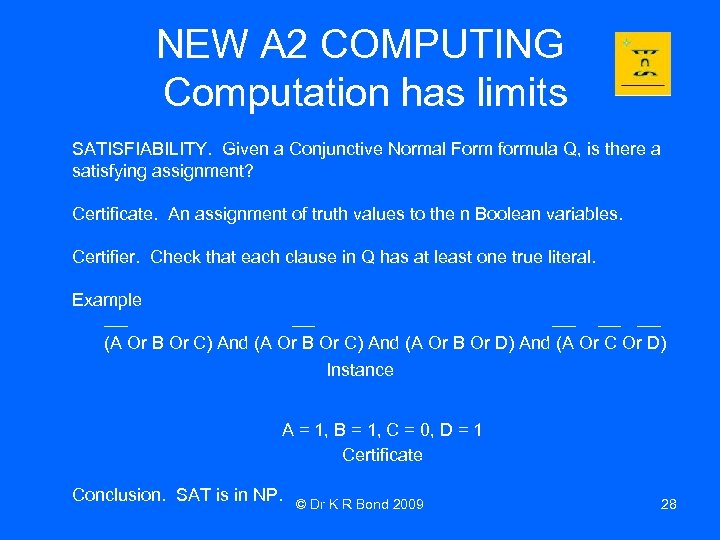NEW A 2 COMPUTING Computation has limits SATISFIABILITY. Given a Conjunctive Normal Form formula Q, is there a satisfying assignment? Certificate. An assignment of truth values to the n Boolean variables. Certifier. Check that each clause in Q has at least one true literal. Example (A Or B Or C) And (A Or B Or D) And (A Or C Or D) Instance A = 1, B = 1, C = 0, D = 1 Certificate Conclusion. SAT is in NP. © Dr K R Bond 2009 28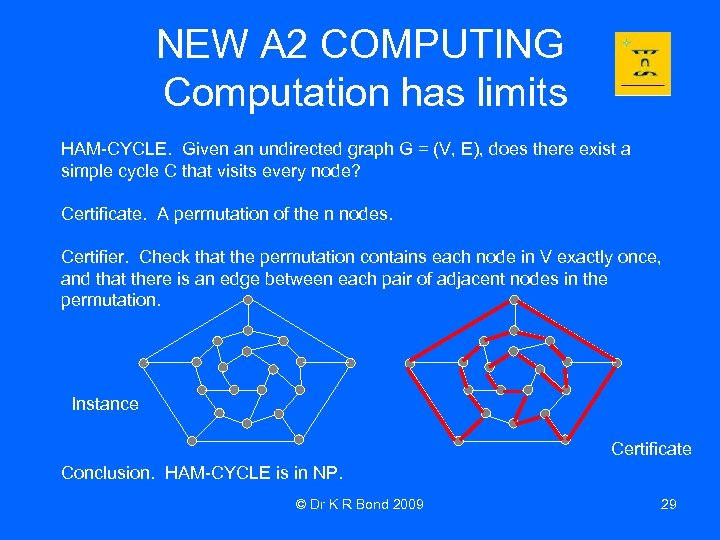NEW A 2 COMPUTING Computation has limits HAM-CYCLE. Given an undirected graph G = (V, E), does there exist a simple cycle C that visits every node? Certificate. A permutation of the n nodes. Certifier. Check that the permutation contains each node in V exactly once, and that there is an edge between each pair of adjacent nodes in the permutation. Instance Certificate Conclusion. HAM-CYCLE is in NP. © Dr K R Bond 2009 29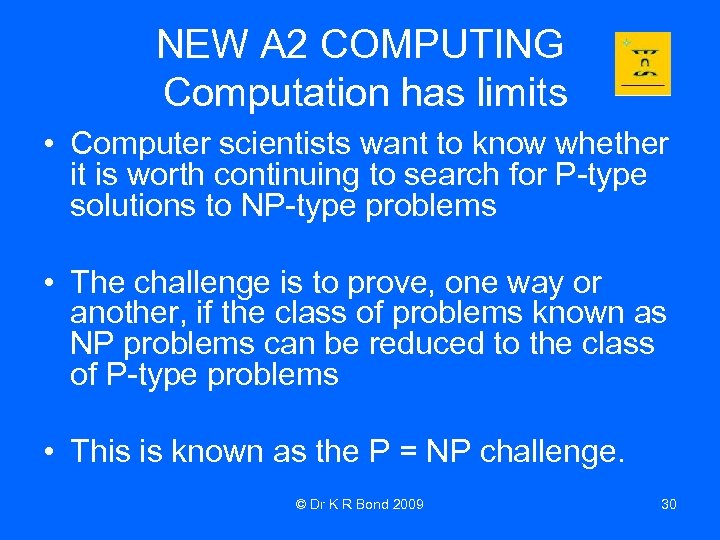NEW A 2 COMPUTING Computation has limits • Computer scientists want to know whether it is worth continuing to search for P-type solutions to NP-type problems • The challenge is to prove, one way or another, if the class of problems known as NP problems can be reduced to the class of P-type problems • This is known as the P = NP challenge. © Dr K R Bond 2009 30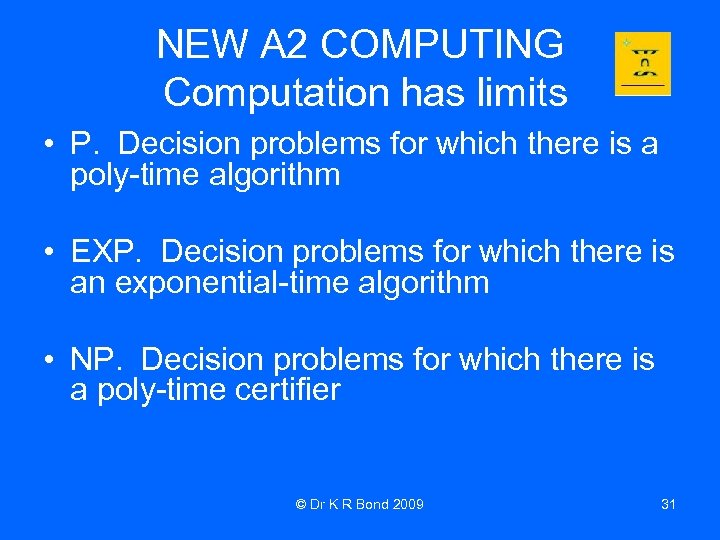NEW A 2 COMPUTING Computation has limits • P. Decision problems for which there is a poly-time algorithm • EXP. Decision problems for which there is an exponential-time algorithm • NP. Decision problems for which there is a poly-time certifier © Dr K R Bond 2009 31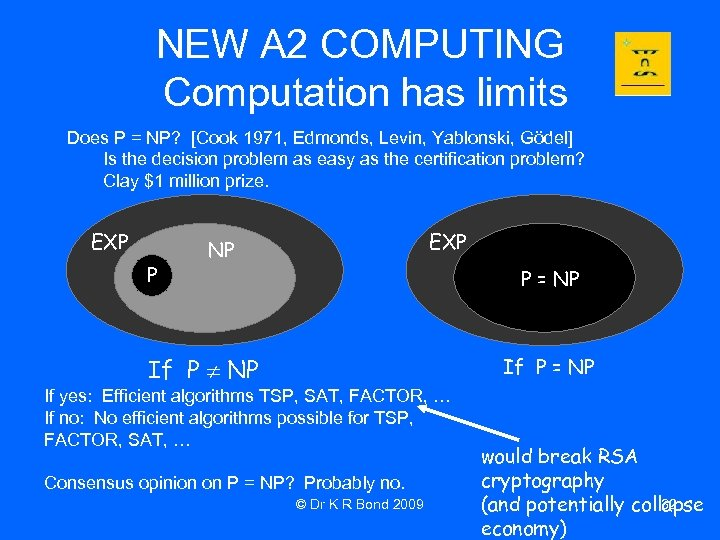NEW A 2 COMPUTING Computation has limits Does P = NP? [Cook 1971, Edmonds, Levin, Yablonski, Gödel] Is the decision problem as easy as the certification problem? Clay \$1 million prize. EXP P EXP NP P = NP If yes: Efficient algorithms TSP, SAT, FACTOR, … If no: No efficient algorithms possible for TSP, FACTOR, SAT, … Consensus opinion on P = NP? Probably no. © Dr K R Bond 2009 would break RSA cryptography 32 (and potentially collapse economy)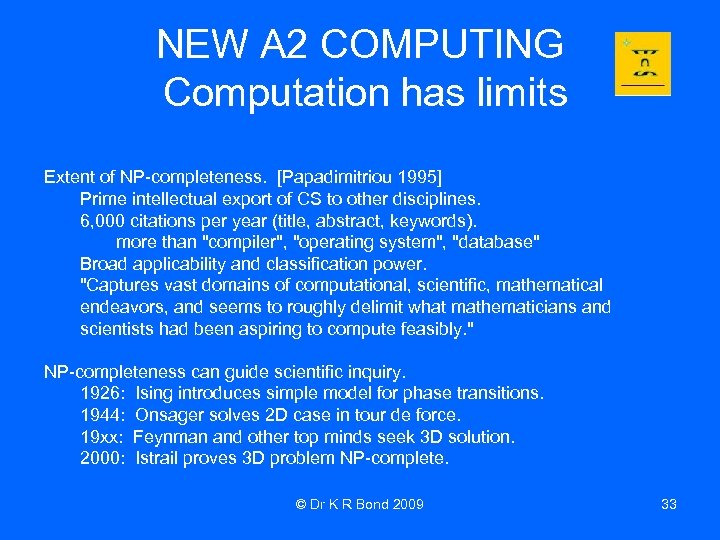NEW A 2 COMPUTING Computation has limits Extent of NP-completeness. [Papadimitriou 1995] Prime intellectual export of CS to other disciplines. 6, 000 citations per year (title, abstract, keywords). more than "compiler", "operating system", "database" Broad applicability and classification power. "Captures vast domains of computational, scientific, mathematical endeavors, and seems to roughly delimit what mathematicians and scientists had been aspiring to compute feasibly. " NP-completeness can guide scientific inquiry. 1926: Ising introduces simple model for phase transitions. 1944: Onsager solves 2 D case in tour de force. 19 xx: Feynman and other top minds seek 3 D solution. 2000: Istrail proves 3 D problem NP-complete. © Dr K R Bond 2009 33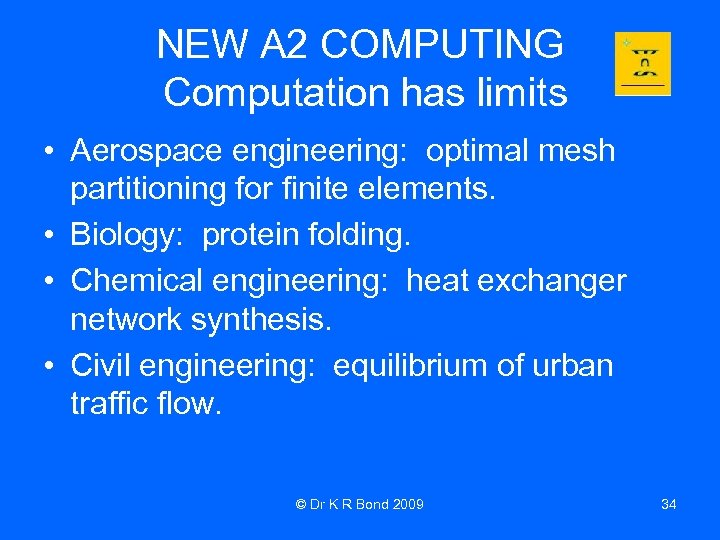NEW A 2 COMPUTING Computation has limits • Aerospace engineering: optimal mesh partitioning for finite elements. • Biology: protein folding. • Chemical engineering: heat exchanger network synthesis. • Civil engineering: equilibrium of urban traffic flow. © Dr K R Bond 2009 34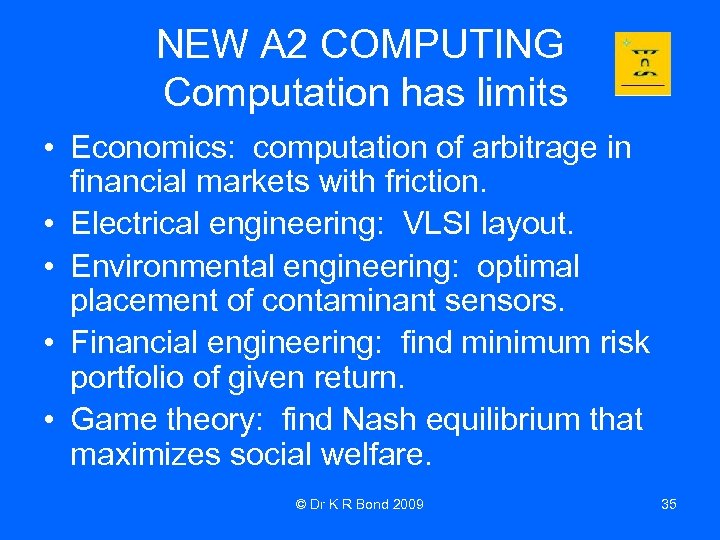NEW A 2 COMPUTING Computation has limits • Economics: computation of arbitrage in financial markets with friction. • Electrical engineering: VLSI layout. • Environmental engineering: optimal placement of contaminant sensors. • Financial engineering: find minimum risk portfolio of given return. • Game theory: find Nash equilibrium that maximizes social welfare. © Dr K R Bond 2009 35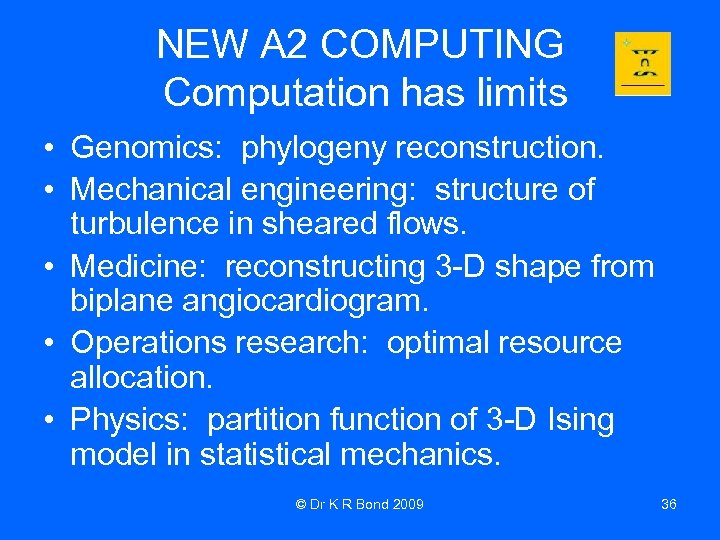NEW A 2 COMPUTING Computation has limits • Genomics: phylogeny reconstruction. • Mechanical engineering: structure of turbulence in sheared flows. • Medicine: reconstructing 3 -D shape from biplane angiocardiogram. • Operations research: optimal resource allocation. • Physics: partition function of 3 -D Ising model in statistical mechanics. © Dr K R Bond 2009 36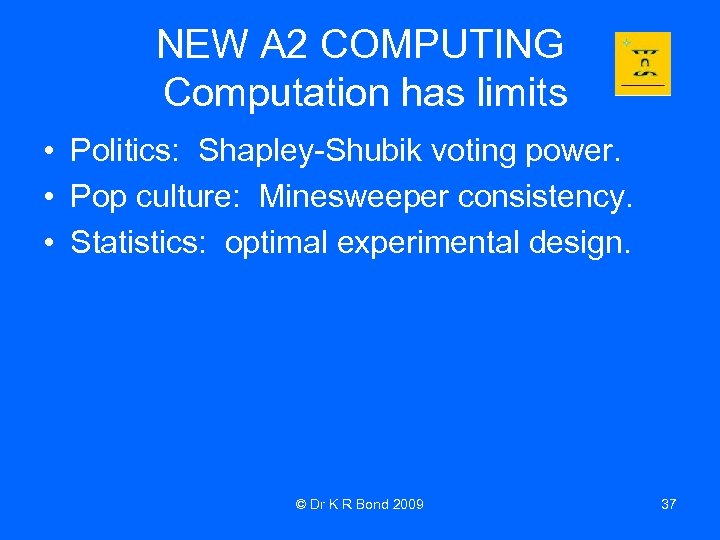NEW A 2 COMPUTING Computation has limits • Politics: Shapley-Shubik voting power. • Pop culture: Minesweeper consistency. • Statistics: optimal experimental design. © Dr K R Bond 2009 37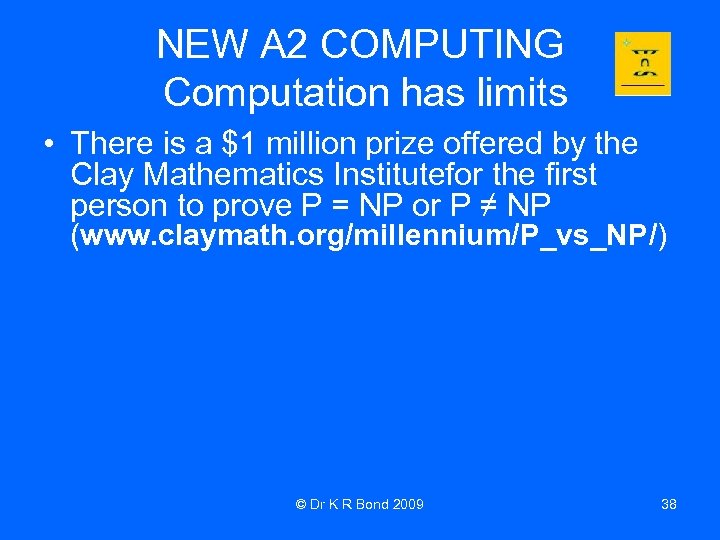NEW A 2 COMPUTING Computation has limits • There is a \$1 million prize offered by the Clay Mathematics Institutefor the first person to prove P = NP or P ≠ NP (www. claymath. org/millennium/P_vs_NP/) © Dr K R Bond 2009 38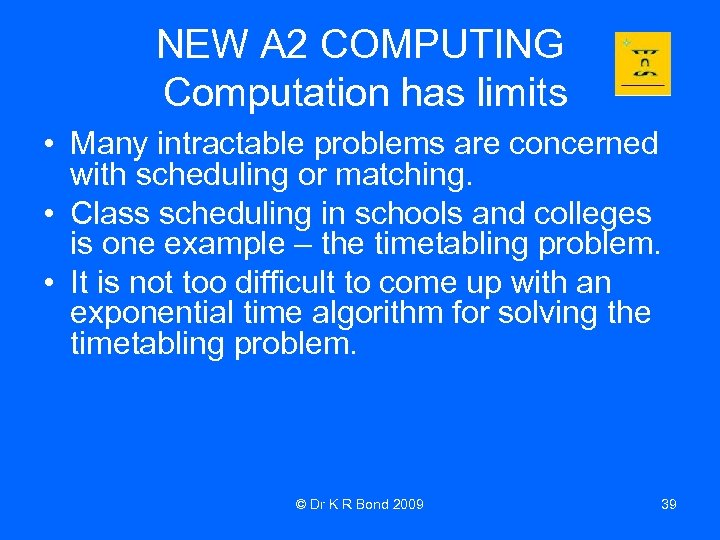NEW A 2 COMPUTING Computation has limits • Many intractable problems are concerned with scheduling or matching. • Class scheduling in schools and colleges is one example – the timetabling problem. • It is not too difficult to come up with an exponential time algorithm for solving the timetabling problem. © Dr K R Bond 2009 39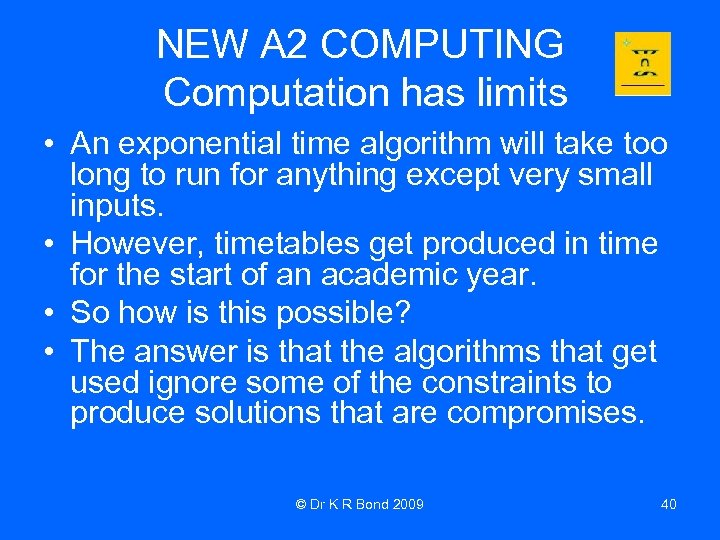NEW A 2 COMPUTING Computation has limits • An exponential time algorithm will take too long to run for anything except very small inputs. • However, timetables get produced in time for the start of an academic year. • So how is this possible? • The answer is that the algorithms that get used ignore some of the constraints to produce solutions that are compromises. © Dr K R Bond 2009 40